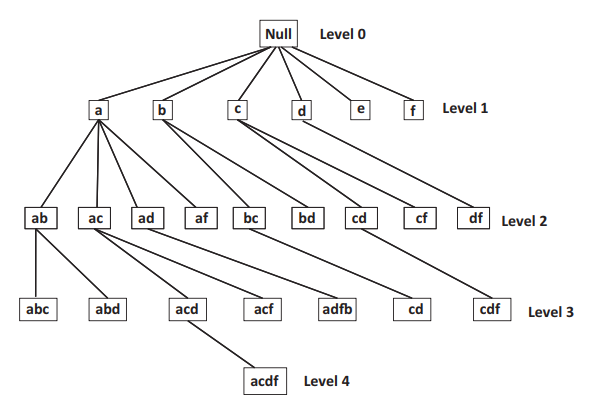# 黄石的时空回环

Let me fly to the 🌕️

### 基本概念

给定一个数据库 D，其中有 T1 ...TN 的交易记录



1. $U \cup V$ 是频繁模式
2. $U \cup V$ 的支持度除以 $U$ 的支持度大于等于 c

• 客户交易分析（调整策略、推荐商品）
• 网页数据挖掘
• 软件 Bug 分析
• 化学和生物分析

• 更高效的算法
• 算法的可扩展性
• 更复杂的数据类型
• 应用研究

### FPM 算法

FPM 算法原型

->从 FP 中生成一个候选模式 P
->if P 在 D 中的支持度 >= s
—>把 P 加入 FP

#### 定义

1. 数据集 T : $T = {T_1,T_2,\cdots,T_n}$，其中 $T_i = {x_1,x_2,\cdots,x_l}$，即数据集中有若干交易记录，每笔记录是物品的集合。
2. 物品集 P : $P \subseteq T_i$，物品集的大小定义为包含的物品的数量。
3. P 的支持度 : 包括 P 的交易的数量。
4. 频繁集 : 若 P 的支持度不小于设定的标准，则 P 是频繁的。

#### Join-Based 算法

Join-Based 的算法通过连接的方式从频繁的 k-patterns 来产生 k+1 的候选。

##### Apriori

Join 连接有 k-1 个元素相同的长度为 k 的频繁模式对。

Apriori 算法

$k:=2$

->通过连接从 $F_k$ 中产生 $k+1$ 的候选集 $C_{k+1}$
->通过子集验证的方法排除一些候选模式
->计算候选集中的支持度来产生 $F_{k+1}$
->$k:=k+i$

##### Apriori 优化
1. AprioriTid: 在第 k 步的时候把每个记录替换成短或空的记录，从而使得子集支持度的计算更高效。但是有时会因为多个候选是记录的子集导致的额外计算开销。
2. AprioriHybrid: 初始迭代的时候不使用 AprioriTid。
##### DHP 算法3

1. 每次迭代的时候修剪候选集。在前一轮计算的时候会保留每个集合的支持度，直接淘汰掉子集支持度不满足要求的候选集。
2. 清理记录使得支持度计算更高效。如果某个项目在 k 的频繁集里面没有出现，那么在 k+1 的频繁集里也不会出现。那这个元素对于后面的支持度计算没有帮助，可以清理掉。

1. 2 元素集合技术的特殊技巧
2. 排除一定满足支持度要求的集合
3. 同时计算多个频繁模式的支持度

#### Tree-Based 算法

Tree-Based 的算法是以集合枚举的概念出发的，候选项可以从词典树中遍历。频繁集的生成等同于建立词典树。而这类树的生长可以是广度优先也可以是深度优先的。##### TreeProjection

TreeProjection 可以说是构建词典树的基本框架，有广度优先和深度优先两种。深度优先的方法有更高效的内存管理。

ExplorePrefix 算法

->把 $P+i$ 加入到频繁模式集中
->从 $T$ 中所有含 $i$ 的数据中构造数据库 $T_i$
->去除 $T_i$ 中词典顺序不大于 i 的所有元素
->ExplorePrefix($T_i$,$s$,$P \cup {i}$)

Itemtidlist
a1,2,3
b1,2,4
c1,2

1. Eclat
2. VIPER

#### 基于后缀的递归算法

##### FP-growth

FP-growth 算法

->对于每条路径中结点的组合 $C$，上报所有的 $C\cup P$ 为频繁项

->对于 $FPT$ 中每一个 $i$
—>$P_i={i}\cup P$
—>上报 $P_i$ 为频繁项
—>从前缀路径中移除不频繁的元素，构建 $FPT_i$
—>if $FPT_i$ 不为空
——->FP-growth($FPT_i$,$P_i$,s)

1. CT-PRO
2. H-Mine

#### 其他优化方法

1. Row Enumeration
2. Pincer-Search

### 参考

1. Frequent Pattern Mining, Ed. Charu Aggarwal and Jiawei Han, Springer, 2014. ↩︎

2. What is Frequent Pattern Mining (Association) and How Does it Support Business Analysis? ↩︎

3. Direct Hashing and Pruning ↩︎

# 本文一共被访问了  次。